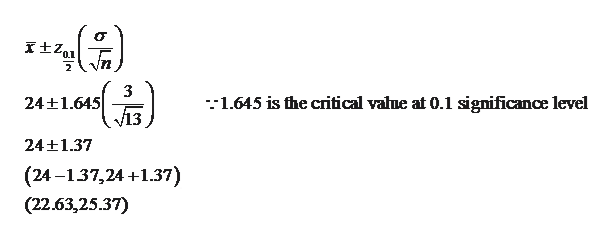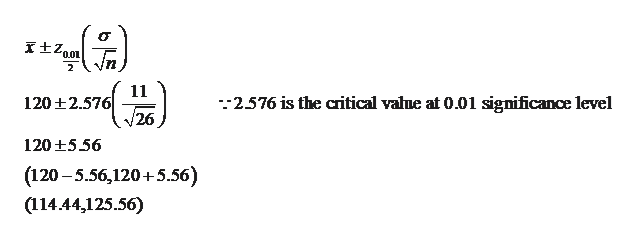Construct a confidence interval for μ assuming that each sample is from a normal population.(a) x⎯⎯x¯ = 24, σ = 3, n = 13, 90 percentage confidence. (Round your answers to 2 decimal places.) The 90% confidence interval is from  _______   to ________ . (b) x⎯⎯x¯ = 120, σ = 11, n = 26, 99 percentage confidence. (Round your answers to 2 decimal places.) The 99% confidence interval is from _______    to _______  . (c) x⎯⎯x¯ = 12.2, σ = 1.3, n = 52, 95 percentage confidence. (Round your answers to 3 decimal places.) The 95% confidence interval is from  ________   to ________ .

Question

Construct a confidence interval for μ assuming that each sample is from a normal population.

(a) x⎯⎯x¯ = 24, σ = 3, n = 13, 90 percentage confidence. (Round your answers to 2 decimal places.)

The 90% confidence interval is from  _______   to ________ .

(b) x⎯⎯x¯ = 120, σ = 11, n = 26, 99 percentage confidence. (Round your answers to 2 decimal places.)

The 99% confidence interval is from _______    to _______  .

(c) x⎯⎯x¯ = 12.2, σ = 1.3, n = 52, 95 percentage confidence. (Round your answers to 3 decimal places.)

The 95% confidence interval is from  ________   to ________ .

Step 1

(a) The 90% confidence interval is:help_outlineImage Transcriptionclosen 2 3 24+1.645 -1.645 is fhe critical valne at 0.1 significance level 24 1.37 (24 -137,24+1.37) (22.63,25.37) fullscreen
Step 2

(b) The 99% confidence inter...help_outlineImage Transcriptionclose0.01 2 11 120 2.576 /26 -2.576 is the critical value at 0.01 significance level 120 5.56 (120-5.56,120+5.56) (114.44,125.56) fullscreen

Want to see the full answer?

See Solution

Want to see this answer and more?

Our solutions are written by experts, many with advanced degrees, and available 24/7

See Solution
Tagged in

Hypothesis Testing